# How to subset from pandas DataFrame?

Pandas DataFrame offer various functions for selecting rows and columns based on column names, column positions, row labels, and row indexes.

Here, we will use pandas `.loc`, `.iloc`, `select_dtypes`, `filter`, NumPy indexing operators `[]`, and attribute operator `.` for selecting rows, columns, and subsets from pandas DataFrame.

## Selecting specific columns from pandas DataFrame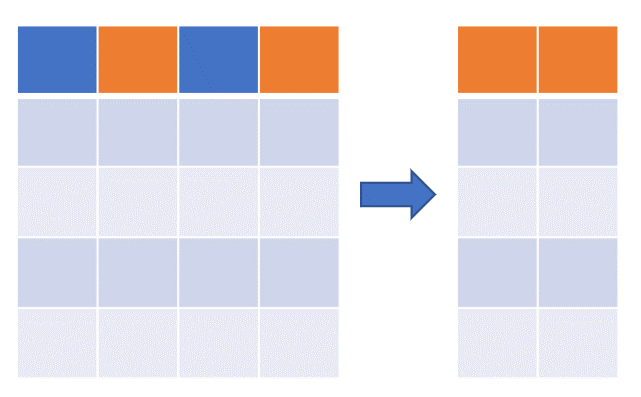Create a pandas DataFrame (you can also import pandas DataFrame from file),

``````import pandas as pd
df = pd.DataFrame({'col1':['A', 'B', 'C', 'D', 'E'], 'col2':[1, 2, 3, 4, 5], 'col3':[0.1, 0.2, 0.3, 0.4, 0.5],
'col4':[True, False, True, True, False]})
df
# output
col1  col2  col3   col4
0    A     1   0.1   True
1    B     2   0.2  False
2    C     3   0.3   True
3    D     4   0.4   True
4    E     5   0.5  False
``````
• There are multiple ways for column selection based on column names (labels) and positions (integer) from pandas DataFrame
• `.loc` indexing is primarily label based and can be used to select columns/rows based on columns/rows names
• `.iloc` indexing is primarily integer based and can be used to select columns/rows based on positions (starting from `0` to `length-1` of the axis i.e. along the rows or columns)

Select any single column,

``````df[['col1']]  # or df.loc[:, 'col1'] or df.col1 or df.iloc[:, 0] or df.filter(items=['col1'])
# output
col1
0    A
1    B
2    C
3    D
4    E
``````

Select multiple columns,

``````df[['col2', 'col4']]
# output
col2   col4
0     1   True
1     2  False
2     3   True
3     4   True
4     5  False

# OTHER WAYS TO SELECT MULTIPLE COLUMNS
# select columns using loc (.loc is primarily label based)
df.loc[:, ['col2', 'col4']]
# output
col2   col4
0     1   True
1     2  False
2     3   True
3     4   True
4     5  False

# select col3 and col4 using iloc (.iloc is primarily integer position based)
df.iloc[:, 2:4]
# output
col3   col4
0   0.1   True
1   0.2  False
2   0.3   True
3   0.4   True
4   0.5  False

# iloc with list
df.iloc[:, [1,3]]
# output
col2   col4
0     1   True
1     2  False
2     3   True
3     4   True
4     5  False
``````

Select multiple columns from list,

``````# select multiple columns which are present in list
col_list = ['col1', 'col2', 'col4']
df[col_list]
# output
col1  col2   col4
0    A     1   True
1    B     2  False
2    C     3   True
3    D     4   True
4    E     5  False

# select multiple columns from list where some columns are present in dataframe and some are not
col_list = ['col1', 'col2', 'col4', 'col5'] # here col5 not present in dataframe
df[df.columns.intersection(col_list)]
# output
col1  col2   col4
0    A     1   True
1    B     2  False
2    C     3   True
3    D     4   True
4    E     5  False
``````

Select multiple columns using column data types using pandas `select_dtypes` function,

``````# select columns containing float values
# column data types can be checked by df.dtypes
df.select_dtypes(include=['float64'])
# output
col3
0   0.1
1   0.2
2   0.3
3   0.4
4   0.5

# select columns containing boolean values
df.select_dtypes(include='bool')
# output
col4
0   True
1  False
2   True
3   True
4  False

# select columns containing numerical values (float and int)
import numpy as np
df.select_dtypes(include=np.number)
# output
col2  col3
0     1   0.1
1     2   0.2
2     3   0.3
3     4   0.4
4     5   0.5
``````

Select columns based on regular expressions using the pandas `filter` function,

``````# select column based on column names
df.filter(items=['col1', 'col4'])
# output
col1   col4
0    A   True
1    B  False
2    C   True
3    D   True
4    E  False

# select all columns where column names starts with col
df.filter(regex='^col', axis=1)
col1  col2  col3   col4
0    A     1   0.1   True
1    B     2   0.2  False
2    C     3   0.3   True
3    D     4   0.4   True
4    E     5   0.5  False

# select all columns where column names ends with character 4
df.filter(regex='4\$', axis=1)
# output
col4
0   True
1  False
2   True
3   True
4  False

# select all columns where column names ends with character 4 or 2
df.filter(regex='4\$|2\$', axis=1)
col2   col4
0     1   True
1     2  False
2     3   True
3     4   True
4     5  False

# select columns which contains the word "col"
df.filter(like='col', axis=1)
# output
col1  col2  col3   col4
0    A     1   0.1   True
1    B     2   0.2  False
2    C     3   0.3   True
3    D     4   0.4   True
4    E     5   0.5  False
``````

## Selecting specific rows from pandas DataFrame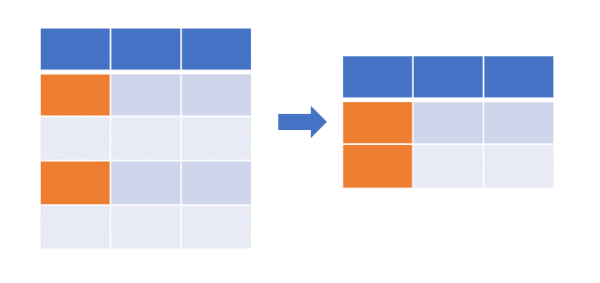Create a pandas DataFrame with index,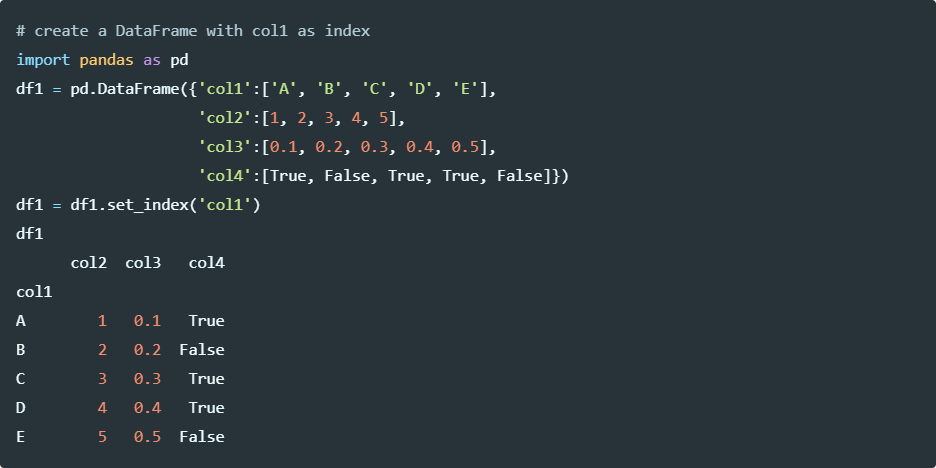Run the code in colab

Selecting rows using `[]` operator, `head`, and `tail` functions,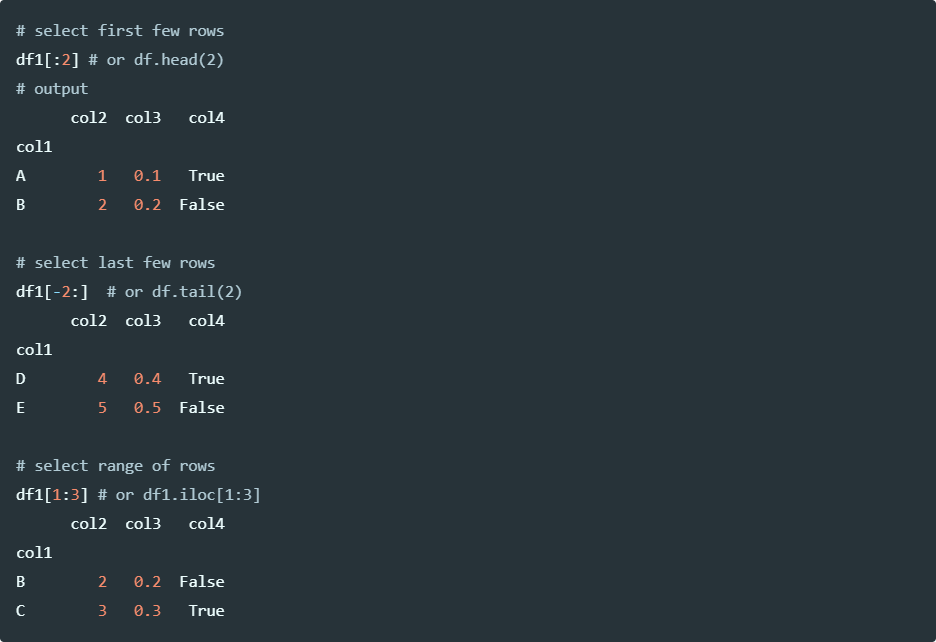Run the code in colab

Selecting rows using index labels,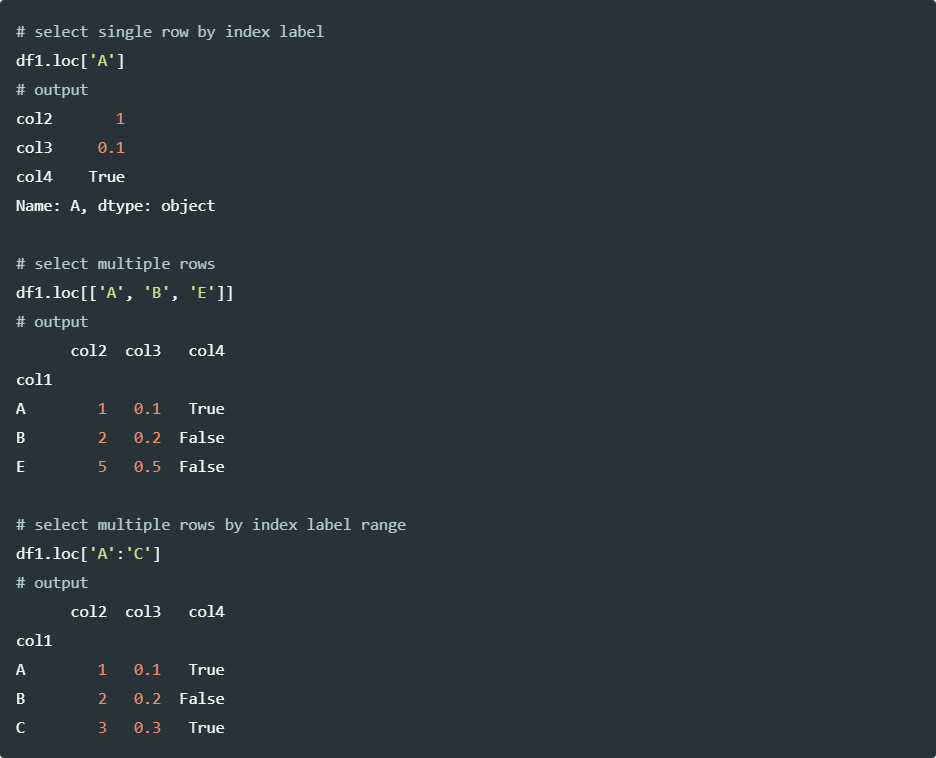Run the code in colab

Select rows based on regex using the pandas `filter` function,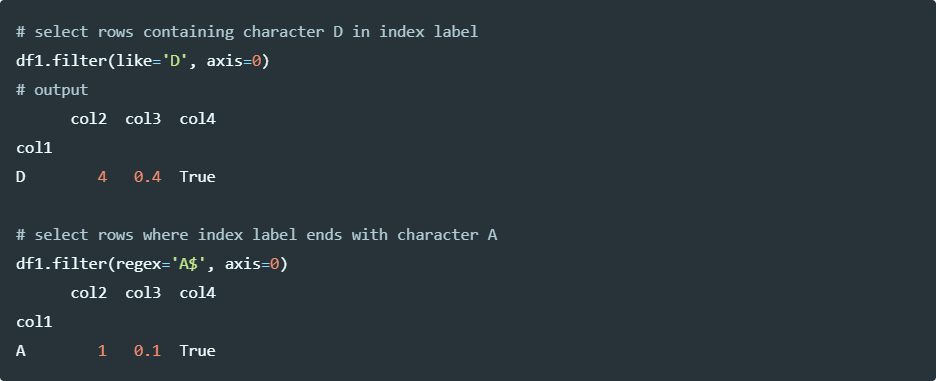Run the code in colab

Select rows based on string regex,

``````# create a DataFrame
import pandas as pd
df2 = pd.DataFrame({'col1':['A', 'B', 'C', 'D', 'E'],
'col2':[1, 2, 3, 4, 5],
'col4':['yes', 'no', 'yes', 'no', 'yes']})
col1  col2 col4
0    A     1  yes
1    B     2   no

# select rows where value of col4 contains the word 'es'
df2[df2.col4.str.contains('es')]
# output
col1  col2 col4
0    A     1  yes
2    C     3  yes
4    E     5  yes
``````

Run the code in colab

Select rows based on column conditions,

``````# select rows where col3 values are greater than 0.2
df1[df1.col3 > 0.2] # or df1.query('col3>0.2') or df1.loc[lambda df1: df1.col3 > 0.2, :]
# output
col2  col3   col4
col1
C        3   0.3   True
D        4   0.4   True
E        5   0.5  False

# select rows where col3 values are greater than 0.2 and col2 value is 4
df1[(df1.col2 == 4) & (df1.col3 > 0.2)]
# output
col2  col3  col4
col1
D        4   0.4  True

# combine AND and OR operator together
df1[((df1.col2 == 4) & (df1.col3 == 0.4)) | ((df1.col2 == 5) & (df1.col4 == True))]
# output
col1  col2  col3  col4
3    D     4   0.4  True
``````

Select rows based on any column value of dataframe matches to any specific value,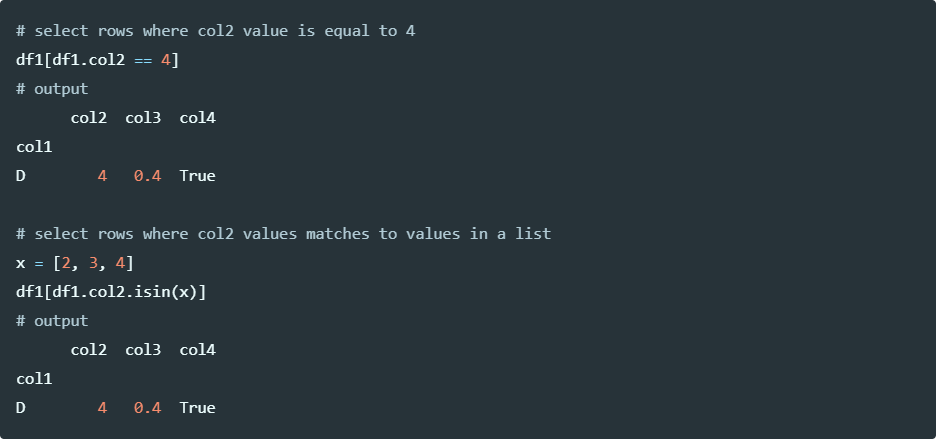Run the code in colab

## Selecting rows and columns from pandas DataFrameSelect rows and columns (a subset of DataFrame) using integer slicing,

``````# select few rows and all columns
# with iloc the start index is included and upper index is excluded
df.iloc[1:3, :]
# output
col1  col2  col3   col4
1    B     2   0.2  False
2    C     3   0.3   True

# select few rows and few columns
df.iloc[1:3, 2:3]
col3   col4
1   0.2  False
2   0.3   True

# select particular dataframe subset using integer list
df.iloc[[1, 3], [0, 3]]
# output
col1   col4
1    B  False
3    D   True
``````

## Reference

If you have any questions, comments or recommendations, please email me at reneshbe@gmail.com